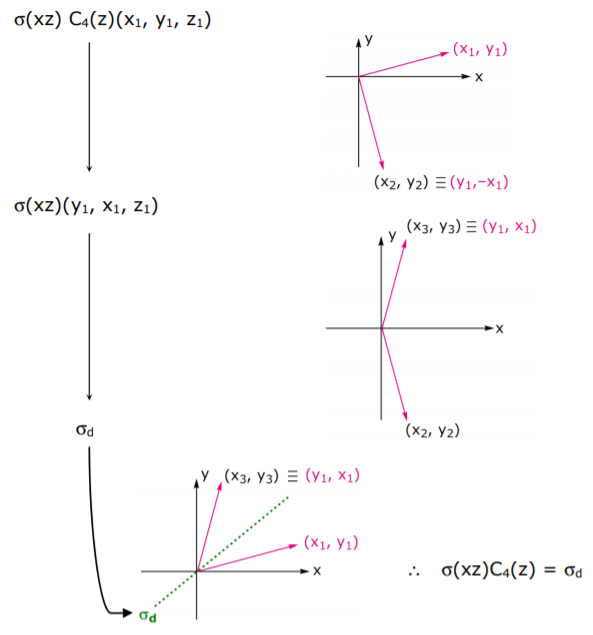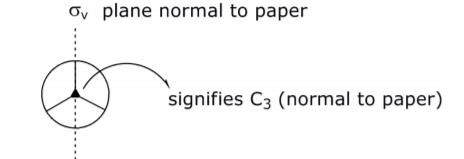# 1.2: Operator Properties and Mathematical Groups

$$\newcommand{\vecs}{\overset { \rightharpoonup} {\mathbf{#1}} }$$ $$\newcommand{\vecd}{\overset{-\!-\!\rightharpoonup}{\vphantom{a}\smash {#1}}}$$$$\newcommand{\id}{\mathrm{id}}$$ $$\newcommand{\Span}{\mathrm{span}}$$ $$\newcommand{\kernel}{\mathrm{null}\,}$$ $$\newcommand{\range}{\mathrm{range}\,}$$ $$\newcommand{\RealPart}{\mathrm{Re}}$$ $$\newcommand{\ImaginaryPart}{\mathrm{Im}}$$ $$\newcommand{\Argument}{\mathrm{Arg}}$$ $$\newcommand{\norm}{\| #1 \|}$$ $$\newcommand{\inner}{\langle #1, #2 \rangle}$$ $$\newcommand{\Span}{\mathrm{span}}$$ $$\newcommand{\id}{\mathrm{id}}$$ $$\newcommand{\Span}{\mathrm{span}}$$ $$\newcommand{\kernel}{\mathrm{null}\,}$$ $$\newcommand{\range}{\mathrm{range}\,}$$ $$\newcommand{\RealPart}{\mathrm{Re}}$$ $$\newcommand{\ImaginaryPart}{\mathrm{Im}}$$ $$\newcommand{\Argument}{\mathrm{Arg}}$$ $$\newcommand{\norm}{\| #1 \|}$$ $$\newcommand{\inner}{\langle #1, #2 \rangle}$$ $$\newcommand{\Span}{\mathrm{span}}$$$$\newcommand{\AA}{\unicode[.8,0]{x212B}}$$

The inverse of A (defined as (A)–1) is B if A ⋅ B = E

For each of the five symmetry operations:

$$( E )^{-1}= E \Longrightarrow( E )^{-1} \cdot E = E \cdot E = E$$
$$(\sigma)^{-1}=\sigma \Longrightarrow(\sigma)^{-1} \cdot \sigma=\sigma \cdot \sigma= E$$
$$(i)^{-1}=i \Longrightarrow(i)^{-1} \cdot i=i \cdot i=E$$
$$\left(C_{n}^{m}\right)^{-1}=C_{n}^{n-m} \Longrightarrow\left(C_{n}^{m}\right)^{-1} \cdot C_{n}^{m}=C_{n}^{n-m} \cdot C_{n}^{m}=C_{n}^{n}=E$$
e.g. $$\left(C_{5}^{2}\right)^{-1}=C_{5}^{3}$$ since $$C_{5}^{2} \cdot C_{5}^{3}=E$$
$$\left(S_{n}^{m}\right)^{-1}=S_{n}^{n-m}(n \text { even }) \Longrightarrow\left(S_{n}^{m}\right)^{-1} \cdot S_{n}^{m}=S_{n}^{n-m} \cdot S_{n}^{m}=S_{n}^{n}=C_{n}^{n} \cdot \sigma_{h}^{n}=E$$
$$\left(S_{n}^{m}\right)^{-1}=S_{n}^{2 n-m}(n \text { odd }) \Longrightarrow\left(S_{n}^{m}\right)^{-1} \cdot S_{n}^{m}=S_{n}^{2 n-m} \cdot S_{n}^{m}=S_{n}^{2 n}=C_{n}^{2 n} \cdot \sigma_{h}^{2 n}=E$$

Two operators commute when A ⋅ B = B ⋅ A

Example: Do C4(z) and σ(xz) commute?… or analyzing with matrix representations,

$$\left[\begin{array}{rrr}0 & 1 & 0 \\ -1 & 0 & 0 \\ 0 & 0 & 1\end{array}\right] \cdot\left[\begin{array}{rrr}1 & 0 & 0 \\ 0 & -1 & 0 \\ 0 & 0 & 1\end{array}\right]=\left[\begin{array}{rrr}0 & -1 & 0 \\ -1 & 0 & 0 \\ 0 & 0 & 1\end{array}\right]$$

C4(z) ⋅ σxz = σd´

Now applying the operations in the inverse order,… or analyzing with matrix representations,

$$\left[\begin{array}{rrr}1 & 0 & 0 \\ 0 & -1 & 0 \\ 0 & 0 & 1\end{array}\right] \cdot\left[\begin{array}{rrr}0 & 1 & 0 \\ -1 & 0 & 0 \\ 0 & 0 & 1\end{array}\right]=\left[\begin{array}{lll}0 & 1 & 0 \\ 1 & 0 & 0 \\ 0 & 0 & 1\end{array}\right]$$

σxz ⋅ C4(z) = σd

\begin{equation}
\therefore \quad C_{4}(z) \sigma(x z)=\sigma_{d}^{\prime} \neq \sigma(x z) C_{4}(z)=\sigma_{d} \Rightarrow \text { so } C_{4}(z) \text { does not commute with } \sigma(x z)
\end{equation}

A collection of operations are a mathematical group when the following conditions are met:

• closure: all binary products must be members of the group
• identity: a group must contain the identity operator
• inverse: every operator must have an inverse
• associativity: associative law of multiplication must hold $(A ⋅ B) ⋅ C = A ⋅ (B ⋅ C$

(note: commutation not required… groups in which all operators do commute are called Abelian)

Consider the operators C3 and σv. These do not constitute a group because identity criterion is not satisfied. Do E, C3, σv form a group? To address this question, a stereographic projection (featuring critical operators) will be used:C3 ⋅ C3 = C3 2 (so C3 2 needs to be included as part of the group)Thus E, C3 and σv are not closed and consequently these operators do not form a group. Is the addition of C3 2 and σv´ sufficient to define a group? In other terms, are there any other operators that are generated by C3 and σv?

… the proper rotation axis, C3:

$$C_{3}$$
$$C _{3} \cdot C _{3}= C _{3}^{2}$$
$$C _{3} \cdot C _{3} \cdot C _{3}= C _{3}^{2} \cdot C _{3}= C _{3} \cdot C _{3}^{2}= E$$
$$C _{3} \cdot C _{3} \cdot C _{3} \cdot C _{3}= E \cdot C _{3}= C _{3}$$

etc.

$$\therefore C _{3}$$ is the generator of $$E , C _{3}$$ and $$C _{3}^{2}$$, note: these three operators form a group

… for the plane of reflection, σv

$$\sigma_{v}$$
$$\sigma_{v} \cdot \sigma_{v}=E$$
$$\sigma_{v} \cdot \sigma_{v} \cdot \sigma_{v}=E \cdot \sigma_{v}=\sigma_{v}$$

etc.
So we obtain no new information here. But there is more information to be gained upon considering C3 and σv. Have already seen that C3 ⋅ σv = σv’ … how about σv ⋅ C3Will discover that no new operators may be generated. Moreover one finds

$$\begin{array}{ccccccc} & E ^{-1} & C _{3}^{-1} & \left( C _{3}^{2}\right)^{-1} & \sigma_{ v }^{-1} & \left(\sigma_{ v }^{\prime}\right)^{-1} & \left(\sigma_{ v }^{\prime \prime}\right)^{-1} \\ \text {inverses } & \downarrow & \downarrow & \downarrow & \downarrow & \downarrow & \downarrow \\ & E & C _{3}^{2} & C _{3} & \sigma_{ v } & \sigma_{ v }^{\prime} & \sigma_{ v }^{\prime \prime}\end{array}$$

The above group is closed, i.e. it contains the identity operator and meets inverse and associativity conditions. Thus the above set of operators constitutes a mathematical group (note that the group is not Abelian).

Some definitions:

Operators C3 and σv are called generators for the group since every element of the group can be expressed as a product of these operators (and their inverses).

The order of the group, designated h, is the number of elements. In the above example, h = 6.

Groups defined by a single generator are called cyclic groups.

Example: C3 → E, C3, C3 2

As mentioned above, E, C3, and C32 meet the conditions of a group; they form a cyclic group. Moreover these three operators are a subgroup of E, C3, C3 2, σv, σv’,σv”. The order of a subgroup must be a divisor of the order of its parent group. (Example hsubgroup = 3, hgroup = 6 … a divisor of 2.)

A similarity transformation is defined as: v -1 ⋅ A ⋅ ν = B where B is designated the similarity transform of A by x and A and B are conjugates of each other. A complete set of operators that are conjugates to one another is called a class of the group.

Let’s determine the classes of the group defined by E, C3, C3 2 , σv, σv’,σv”… the analysis is facilitated by the construction of a multiplication table

$\begin{array}{l|llllll} & E & C _{3} & C _{3}^{2} & \sigma_{ v } & \sigma_{ v }^{\prime} & \sigma_{ v }^{\prime \prime} \\ \hline E & E & C _{3} & C _{3}^{2} & \sigma_{ v } & \sigma_{ v }^{\prime} & \sigma_{ v }^{\prime \prime} \\ C _{3} & C _{3} & C _{3}^{2} & E & \sigma_{ v }^{\prime} & \sigma_{ v }^{\prime \prime} & \sigma_{ v } \\ C _{3}^{2} & C _{3}^{2} & E & C _{3} & \sigma_{ v }^{\prime \prime} & \sigma_{ v } & \sigma_{ v }^{\prime} \\ \sigma_{ v } & \sigma_{ v } & \sigma_{ v }^{\prime \prime} & \sigma_{ v }^{\prime} & E & C _{3}^{2} & C _{3} \\ \sigma_{ v }^{\prime} & \sigma_{ v }^{\prime} & \sigma_{ v } & \sigma_{ v }^{\prime \prime} & C _{3} & E & C _{3}^{2} \\ \sigma_{ v }^{\prime \prime} & \sigma_{ v }^{\prime \prime} & \sigma_{ v }^{\prime} & \sigma_{ v } & C _{3}^{2} & C _{3} & E \end{array}$

may construct easily using stereographic projections

$$E ^{-1} \cdot C _{3} \cdot E = E \cdot C _{3} \cdot E = C _{3}$$
$$C _{3}^{-1} \cdot C _{3} \cdot C _{3}= C _{3}^{2} \cdot C _{3} \cdot C _{3}= E \cdot C _{3}= C _{3}$$
$$\left( C _{3}^{2}\right)^{-1} \cdot C _{3} \cdot C _{3}^{2}= C _{3} \cdot C _{3} \cdot C _{3}^{2}= C _{3} \cdot E = C _{3}$$
$$\sigma _{ v }^{-1} \cdot C _{3} \cdot \sigma_{ v }=\sigma_{ v } \cdot C _{3} \cdot \sigma_{ v }=\sigma_{ v } \cdot \sigma_{ v }^{\prime}= C _{3}^{2}$$
$$\left(\sigma_{ v }^{\prime}\right)^{-1} \cdot C _{3} \cdot \sigma_{ v }^{\prime}=\sigma_{ v }^{\prime} \cdot C _{3} \cdot \sigma_{ v }^{\prime}=\sigma_{ v }^{\prime} \cdot \sigma_{ v }^{\prime \prime}= C _{3}^{2}$$
$$\left(\sigma_{ v }^{\prime \prime}\right)^{-1} \cdot C _{3} \cdot \sigma_{ v }^{\prime \prime}=\sigma_{ v }^{\prime \prime} \cdot C _{3} \cdot \sigma_{ v }^{\prime \prime}=\sigma_{ v }^{\prime \prime} \cdot \sigma_{ v }= C _{3}^{2}$$

∴ C3 and C3 2 from a class

Performing a similar analysis on σv will reveal that σv, σv’ and σv’’ form a class and E is in a class by itself. Thus there are three classes:

$$E ,\left( C _{3}, C _{3}^{2}\right),\left(\sigma_{ v }, \sigma_{ v }^{\prime}, \sigma_{ v }^{\prime \prime}\right)$$

Additional properties of transforms and classes are:

• no operator occurs in more than one class
• order of all classes must be integral factors of the group’s order
• in an Abelian group, each operator is in a class by itself.

This page titled 1.2: Operator Properties and Mathematical Groups is shared under a CC BY-NC-SA 4.0 license and was authored, remixed, and/or curated by Daniel Nocera via source content that was edited to the style and standards of the LibreTexts platform; a detailed edit history is available upon request.